### Vol 17, No 10 (2017) / Liu

Strongly screened electron capture rates of chromium isotopes in presupernova evolution

# Strongly screened electron capture rates of chromium isotopes in presupernova evolution

Liu Jing-Jing1, , Peng Qiu-He2, Hao Liang-Huan1, Kang Xiao-Ping1, Liu Dong-Mei1

College of Marine Science and Technology, Hainan Tropical Ocean University, Sanya 572022, China
Department of Astronomy, Nanjing University, Nanjing 210093, China

† Corresponding author. E-mail: liujingjing68@126.com

Abstract: Abstract

Taking into account the effect of electron screening on electron energy and electron capture threshold energy, by using the method of Shell-Model Monte Carlo and random phase approximation theory, we investigate the capture rates of chromium isotopes with strong electron screening according to the linear response theory screening model. Strong screening rates can decrease by about 40.43% (e.g., for 60Cr at T 9 = 3.44, Y e = 0.43). Our conclusions may be helpful to researches on supernova explosions and related numerical simulation methods.

Keywords: nuclear reactions;electron capture;supernovae

## 1 Introduction

At the presupernova stage, beta decay and electron capture (EC) on some neutron-rich nuclei may play important roles in determining the hydrostatic core structure of massive presupernova stars, thereby affecting their subsequent evolution during the gravitational collapse and supernova explosion phases (e.g., Dean et al. ; Caurier et al. ; Juodagalvis et al. ; Liu , ; Liu et al. ; Liu & Gu ; Liu et al. ). For example, beta decay (EC) strongly influences the time rate of change of the lepton fraction (e.g., the time rate of change of electron fraction e) by increasing (decreasing) the number of electrons. Some isotopes of iron, chromium and copper can also make a substantial contribution to the overall changes in lepton fraction (e.g., e), electron degeneracy pressure and entropy of the stellar core during its very late stage of evolution. Many of these nuclei can be appropriately tracked in the reaction network of stellar evolution calculations. The lepton fraction (e.g., e) is bound to lead to an unstoppable process of gravitational collapse and supernova explosion.

Some research has shown that EC in iron group nuclei (e.g., iron and chromium isotopes) is a very important and dominant process in supernova explosions (e.g., Aufderheide et al. , ; Dean et al. ; Heger et al. ). In the process of presupernova evolution, chromium isotopes are a very important and crucial radionuclide. Aufderheide et al. () investigated EC and beta decay for these nuclei in detail in presupernova evolution. They found that the EC rates of these chromium isotopes can be of significant astrophysical importance by controlling the electronic abundance. Heger et al. () also discussed weak-interaction rates for some iron group nuclei by employing shellmodel calculations in presupernova evolution. They found that EC rates on iron group nuclei would be crucial for decreasing the electronic abundance (Y e) in stellar matter.

On the other hand, in the process of presupernova evolution in massive stars, the Gamow-Teller (GT) transitions of isotopes of chromium play a consequential role. Some studies have shown that β-decay and EC rates of chromium isotopes significantly affect the time rate of change of lepton fraction ( e). For example, Nabi et al. () detailed the GT strength distributions, e and neutrino energy loss rates for chromium isotopes due to weak interactions in stellar matter.

However, their works did not discuss the problem of how strong electron screening (SES) would effect EC. What role does EC play in stellar evolution? How does SES influence the EC reaction at high density and temperature? In order to accurately calculate the EC rates and screening correction for numerical simulations of supernova explosions, in this paper we will discuss this problem in detail.

Based on the linear response theory model (LRTM) and random phase approximation (RPA), we study strongly screened EC rates of chromium isotopes in astrophysical environments by using the Shell-Model Monte Carlo (SMMC) method. In the next section, we discuss the methods used for EC in stellar interiors in the cases with and without SES. Section 3 will present some numerical results and discussions. Conclusions follow in Section 4.

## 2 EC rates in the process of stellar core collapse

2.1 EC Rates in the Case without SES

For nucleus (Z, A), we calculate the stellar EC rates, which are given by a sum over the initial parent states i and the final daughter states f at temperature T. This expression is written as (e.g., Fuller et al. , )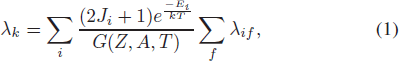where J i is the spin and E i is excitation energies of the parent states. The nuclear partition function G(Z, A, T) has been discussed by Aufderheide et al. (, ). λ if denotes the rates from one of the initial states to all possible final states.

Based on the theory of RPA, the EC rates are closely related to cross section σ ec, and can written as (e.g., see detailed discussions in Dean et al. ; Caurier et al. ; Juodagalvis et al. )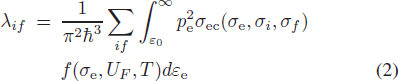where ε 0 = max(Q if , 1). The incoming electron momentum is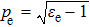, ε e is the electron energy, the electron chemical potential is given by UF and T is the electron temperature. The energies and momenta are in units of m e c 2 and m e c respectively (m e is the electron mass and c is the speed of light).

The electron chemical potential is obtained by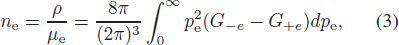where μ e and ρ are the average molecular weight and the density in g cm−3, respectively.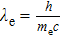is the Compton wavelength,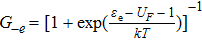and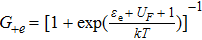are the electron and positron distribution functions respectively, and k is the Boltzmann constant. The phase space factor is defined as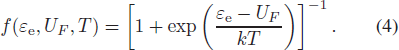According to energy conservation, the electron, proton and neutron energies are related to the neutrino energy, and the Q-value for the capture reaction is (Cooperstein & Wambach )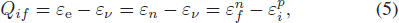so we have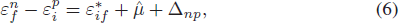where εv is neutrino energy,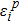is the energy of an initial proton single particle state and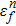is the energy of a neutron single particle state.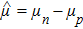and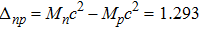MeV are the chemical potential and mass difference between a neutron and proton in the nucleus, respectively.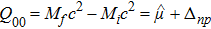, and the masses of the parent nucleus and daughter nucleus correspond to Mi and Mf respectively;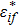is the excitation energies for the daughter nucleus at zero temperature.

The total cross section in the process of EC reaction is given by (e.g., Dean et al. ; Caurier et al. ; Juodagalvis et al. )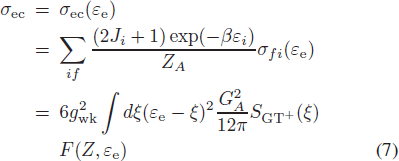where g wk = 1.1661 × 10−5 GeV−2 is the weak coupling constant and GA = 1.25. F(Z, ε e) is the factor for Coulomb wave correction.

The total amount of GT strength is S GT +, which is calculated by summing over a complete set from an initial state to final states. The response function RA (τ) of an operator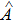at an imaginary time τ is calculated by using the method of SMMC. Thus, RA (τ) is given by (e.g., Dean et al. ; Juodagalvis et al. )

The strength distribution is related to RA (τ) by a Laplace transform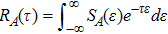and is given by (e.g., Dean et al. ; Caurier et al. ;Juodagalvis et al. )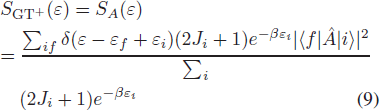where ε is the energy transfer within the parent nucleus, the S GT +(ε) is in units of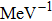,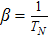and TN is the nuclear temperature.

For degenerate relativistic electron gas, the EC rates in the case without SES are given by (e.g., Dean et al. ; Caurier et al. ; Juodagalvis et al. )The p 0 is defined as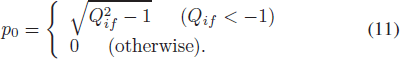2.2 EC Rates in the Case with SES

In 2002, based on the LRTM for relativistic degenerate electrons, Itoh et al. () discussed the effect of screening potential on EC. The electron is strongly degenerate in our considered regime of density-temperature. This condition is expressed as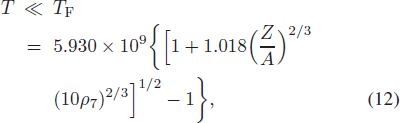where T F and ρ 7 are the electron Fermi temperature and density (in units of 107 g cm−3).

For a relativistically degenerate electron liquid, Jancovici () studied the static longitudinal dielectric function. Taking into account the effect of strong screening, the electron potential energy is written as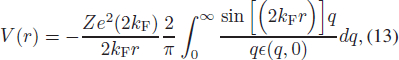where ε(q, 0) is Jancovici’s static longitudinal dielectric function and k F is the electron Fermi wave-number.

The screening potential for relativistic degenerate electrons from linear response theory is written as (Itoh et al. )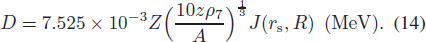Itoh et al. () discussed the parameters J(r s,R), r s and R in detail. Equation (14) is fulfilled in a presupernova environment and is satisfied for

The screening energy is sufficiently high that we cannot neglect its influence at high density when electrons are strongly screened. The electron screening will make electron energy decrease from ε to ε′ = εD in the process of EC. Meanwhile, the screening relatively increases threshold energy from ε 0 to ε s = ε 0 + D for EC. So, the EC rates in SES are given by (e.g., Juodagalvis et al. ; Liu )

The nuclear binding energy will increase due to interactions with the dense electron gas in the plasma. The effective nuclear Q-value (Qif ) will change at high density due to the influence of the charge dependence on this binding. When we take the effect of SES into account, the EC Q-value will increase by (Fuller et al. )

Therefore, the Q-value of EC increases from Qif to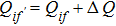. The ε s is defined as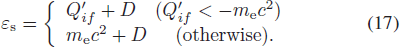We define the screening enhancement factor C to enable a comparison of the results as follows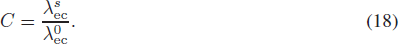## 3 Numerical Calculations Of EC Rates and Discussion

The influences of SES on EC rates for these chromium isotopes at some typical astrophysical conditions are shown in Figure 1. Note that the no SES and SES rates correspond to solid and dotted lines respectively. We give details about the EC process according to the SMMC method, especially for the contribution to EC due to the GT transition. For a given temperature, the EC rates increase by more than six orders of magnitude as the density increases. Based on the proton-neutron quasiparticle RPA (pn-QRPA) model, Nabi & Klapdor-Kleingrothaus (NKK) also investigated the EC rates in detail in the case without SES. Their results also showed that density strongly influences the EC rates for a given temperature. For example, the EC rate for 61Cr increases from 6.3096 × 10−23 s−1 to 3.71535 × 102 s−1 when the density changes from 107 g cm−3 to 1011 g cm−3 at T9 = 3 (see the detailed discussions in Nabi & Klapdor-Kleingrothaus ). Under the same conditions, the Fuller, Fowler & Newman rate for 60Cr increases from 8.3946×10−26 s−1 to 1.2388×103 s−1 (see Fuller et al. ). These studies demonstrate that the stellar weak rates play a key role in the dynamics of core collapse calculations and stellar numerical simulation.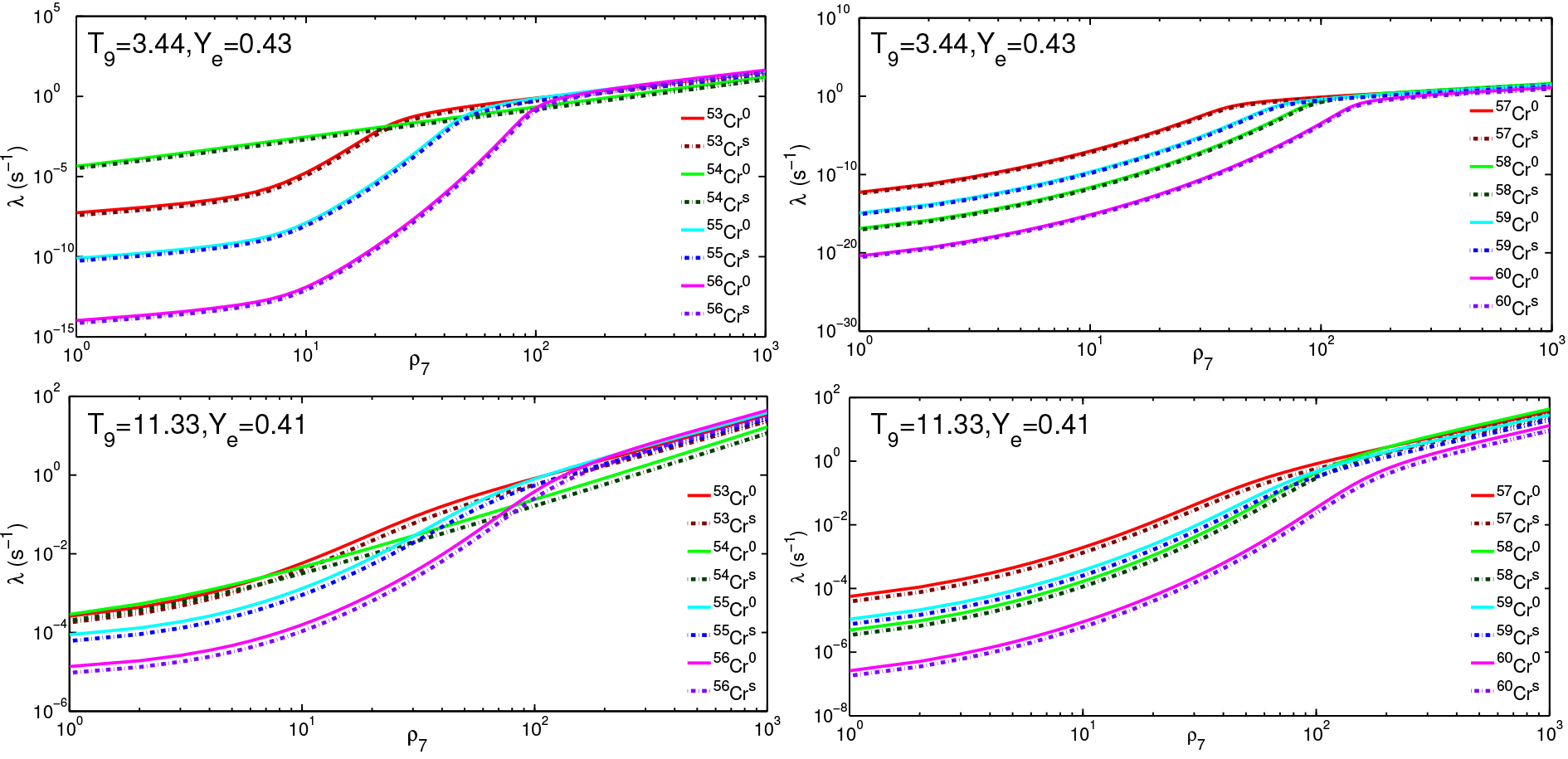Fig. 1 The no SES and SES rates correspond to solid and dotted lines respectively for chromium isotopes as a function of density ρ 7 at temperatures of T 9 = 3.44, Y e = 0.43 and T 9 = 11.33, Y e = 0.41.

According to our calculations, the GT transition EC reaction may not be the dominant process at lower temperature. On the other hand, the higher the temperature is, the larger the electron energy, the larger the density and the higher the electron Fermi energy become. Therefore, a lot of electrons join in the EC reaction and the GT transition would be very active and be the dominant contribution to the total EC rates. Figure 1 displays the screening rates and no screening rates, which correspond to solid and dotted lines respectively as a function of density. We find that the screening rates are commonly lower than no screening rates.

The GT strength distributions play a significant role in supernova evolution. However, the GT+ transitions are addressed only qualitatively in presupernova simulations because of insufficient experimental information. The general rule is that the energy for the daughter ground state is parameterized phenomenologically by assuming the GT+ strength resides in a single resonance. Charge exchange reactions (n, p) and (p, n) would, if obtainable, supply us with plenty of experimental information. However, any available experimental GT+ strength distributions for these nuclei cannot be obtained except for theoretical calculations.

Table 1 presents a comparison of our results by SMMC for total strength, centroid and width of calculated GT strength distributions with those of NKK (Nabi et al. ) for EC of 53−60Cr. Our results of calculated GT strength distributions are higher than those of NKK.

ΣB(GT)+ E+(MeV) Width+ (MeV)
Nuclide NKK SMMC NKK SMMC NKK SMMC
53Cr 0.51 0.5625 6.21 6.334 2.72 2.813
54Cr 1.95 2.2340 2.88 2.912 3.32 3.406
55Cr 0.39 0.4130 4.06 4.126 3.47 3.675
56Cr 1.31 1.3326 1.77 1.791 2.14 2.366
57Cr 0.25 0.2740 5.21 5.267 2.84 2.972
58Cr 0.82 0.8411 1.57 1.605 2.49 2.560
59Cr 0.24 0.2520 1.26 1.302 2.24 2.272
60Cr 0.39 0.4012 3.03 3.201 4.99 5.017

Table 1Comparison of our results by SMMC for total strength, centroid and width of calculated GT strength distributions with those of NKK (Nabi et al. ) for EC of 53−60Cr..

Based on pn-QRPA theory, NKK analyzed the nuclear excitation energy distribution by taking into consideration particle emission processes. They calculated a stronger GT strength distribution from these excited states compared to those assumed using Brink’s hypothesis. However, in their works, they only discussed the low angular momentum states. By using the method of SMMC, the GT intensity distribution is discussed in detail and an average value of the distribution is actually adopted in our paper.

Values for the screening factor C are plotted as a function of ρ 7 in Figure 2. Due to SES, the rates decrease by about 40.43%. The lower the temperature, the larger the effect of SES on EC rates is. This is due to the fact that SES mainly decreases the number of higher energy electrons which can actively join in the EC reaction. Moreover, the SES can also make the EC threshold energy increase greatly. As a matter of fact, SES will strongly weaken the progress of EC reactions. One can also find that the screening factor almost tends to the same value at higher density and it is not dependent on the temperature or density. The reason is that at higher density the electron energy is mainly determined by its Fermi energy, which is strongly decided by density.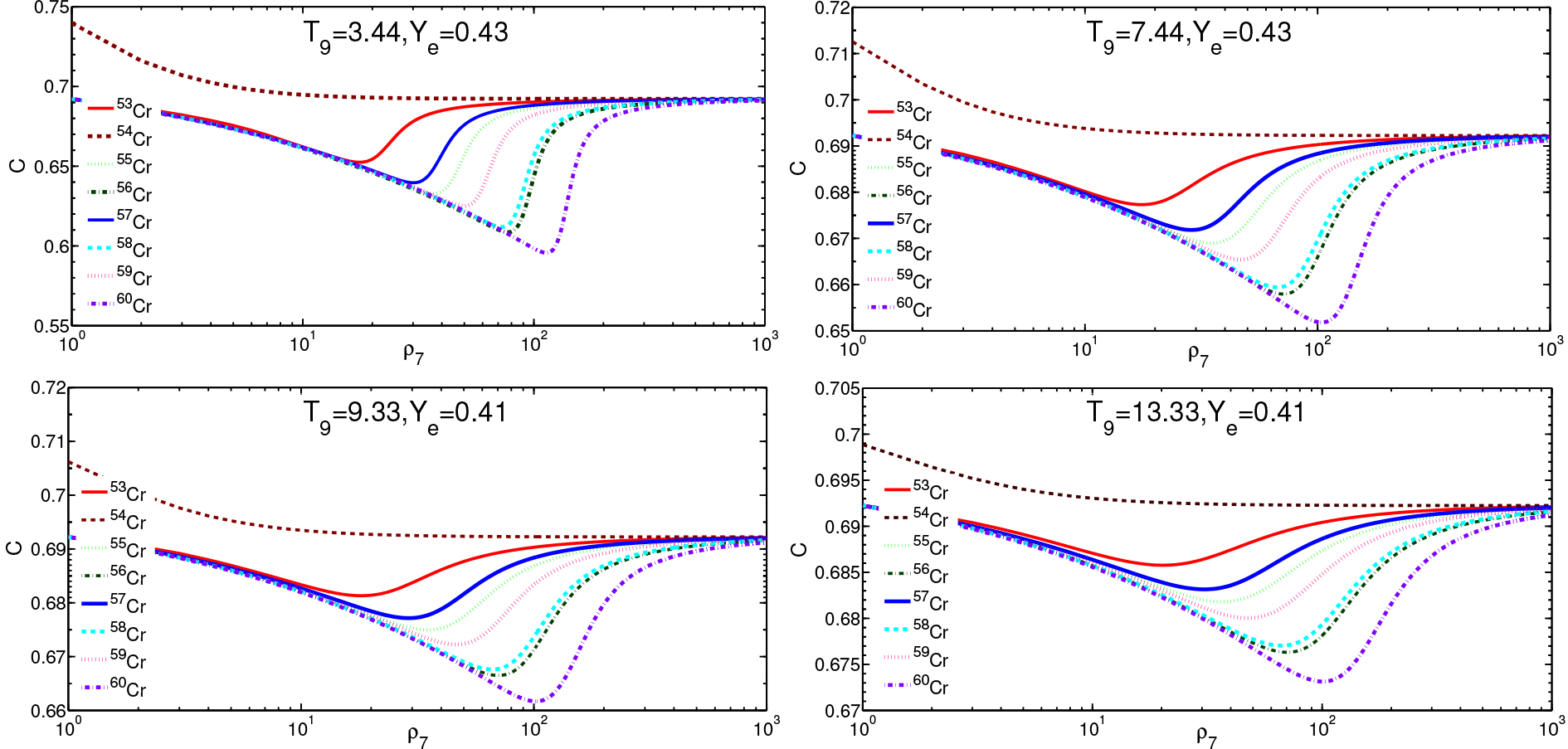Fig. 2 The SES enhancement factor C for chromium isotopes as a function of the density ρ 7 at temperatures of T 9 = 3.44, 7.44, Y e = 0.43 and T 9 = 9.33, 11.33, Y e = 0.41. Four different line styles correspond to 53−56Cr. The same line styles also correspond to 57−60Cr. But the latter is far coarser than the former.

Table 2 shows details about the numerical calculations of minimum values for screening factor C min. One finds that the EC rates decrease greatly due to SES. For instance, from Table 2 that provides values for the factor C min, the rates decrease about 34.75%, 30.77%, 36.92%, 39.07%, 35.98%, 38.81%, 37.50% and 40.43% for 53−60Cr at T 9 = 3.44, Y e = 0.43. This is due to the fact that the SES mainly decreases the number of higher energy electrons, which can actively join in EC reactions. On the other hand, the screening of nuclear electric charges with a high electron density means a short screening length, which results in a lower enhancement factor from Coulomb wave correction. However, even a relatively short electric charge screening length will not have much effect on the overall rate due to the weak interaction being effectively a contact potential. A bigger effect is that electrons are bound in the plasma.

T 9 = 3.44, Y e = 0.43 T 9 = 7.44, Y e = 0.43 T 9 = 9.33, Y e = 0.41 T 9 = 13.33, Y e = 0.41
Nuclide ρ7 C min ρ7 C min ρ7 C min ρ7 C min
53Cr 18 0.6525 19 0.6774 19 0.6813 20 0.6858
54Cr 62 0.6923 65 0.6924 66 0.6924 67 0.6924
55Cr 38 0.6308 37 0.6690 36 0.6750 37 0.6818
56Cr 81 0.6093 72 0.6580 71 0.6665 71 0.6763
57Cr 32 0.6402 30 0.6719 31 0.6772 33 0.6832
58Cr 74 0.6119 69 0.6594 67 0.6676 67 0.6770
59Cr 50 0.6250 47 0.6654 49 0.6723 48 0.6800
60Cr 115 0.5957 106 0.6518 104 0.6617 99 0.6731

Table 2 The Minimum Values of Strong Screening Factor C for Some Typical Astronomical Conditions when 1≤7≤103

Synthesizing the above analysis, the effects of charge screening on nuclear physics (e.g., EC and beta decay) come at least from the following factors. First, the screening potential will change the electron Coulomb wave function in nuclear reactions. Second, the electron screening potential decreases the energy of incident electrons joining in the capture reactions. Third, the electron screening increases the energy of atomic nuclei (i.e., increases the single particle energy) in nuclear reactions. Finally, the electron screening effectively decreases the number of higher-energy electrons, whose energy is more than the threshold of the capture reaction. Therefore, screening relatively increases the threshold needed for capture reactions and decreases the capture rates.

## 4 Concluding remarks

In this paper, based on the theory of RPA and LRTM and by using the method of SMMC, we investigate the EC rates in SES. The EC rates increase greatly by more than six orders of magnitude as the density increases. On the other hand, by taking into account the influence of SES on the energy of incident electrons and threshold energy of EC, the EC rates decrease by ∼ 40.43%.

ECs play an important role in the dynamic process of the collapsing core of a massive star. It is a main parameter for supernova explosion and stellar collapse. SES strongly influences EC and may influence the cooling rate and evolutionary timescale of stellar evolution. Thus, the conclusions we obtained may have a significant influence on further research of supernova explosions and related numerical simulations.

 Aufderheide M. B. Brown G. E. Kuo T. T. S. Stout D. B. Vogel P. 1990 ApJ 362 241 Aufderheide M. B. Fushiki I. Woosley S. E. Hartmann D. H. 1994 ApJS 91 389 Caurier E. Langanke K. Martínez-Pinedo G. Nowacki F. 1999 Nuclear Physics A 653 439 Cooperstein J. Wambach J. 1984 Nuclear Physics A 420 591 Dean D. J. Langanke K. Chatterjee L. Radha P. B. Strayer M. R. 1998 Phys. Rev. C 58 536 Fuller G. M. Fowler W. A. Newman M. J. 1980 ApJS 42 447 Fuller G. M. Fowler W. A. Newman M. J. 1982 ApJS 48 279 Heger A. Woosley S. E. Martínez-Pinedo G. Langanke K. 2001 ApJ 560 307 Itoh N. Tomizawa N. Tamamura M. Wanajo S. Nozawa S. 2002 ApJ 579 380 Jancovici B. 1962 Il Nuovo Cimento (1955-1965) 25 428 Juodagalvis A. Langanke K. Hix W. R. Martínez-Pinedo G. Sampaio J. M. 2010 Nuclear Physics A 848 454 Liu J.-J. 2013 MNRAS 433 1108 Liu J.-J. 2014 MNRAS 438 930 Liu J.-J. Gu W.-M. 2016 ApJS 224 29 Liu J.-J. Kang X.-P. Hao L.-H. et al. 2016 RAA (Research in Astronomy and Astrophysics) 16 174 Liu J.-J. Peng Q.-H. Liu D.-M. 2017 arXiv:1701.05771 Nabi J. Klapdor-Kleingrothaus H. V. 1999 nuclth/9907115 Nabi J.-U. Shehzadi R. Fayaz M. 2016 Ap & SS 361 95
Cite this article: Liu Jing-Jing, Peng Qiu-He, Hao Liang-Huan, Kang Xiao-Ping, Liu Dong-Mei. Strongly screened electron capture rates of chromium isotopes in presupernova evolution. Cancer Biol Med. 2017; 10:107.

### Refbacks

• There are currently no refbacks.